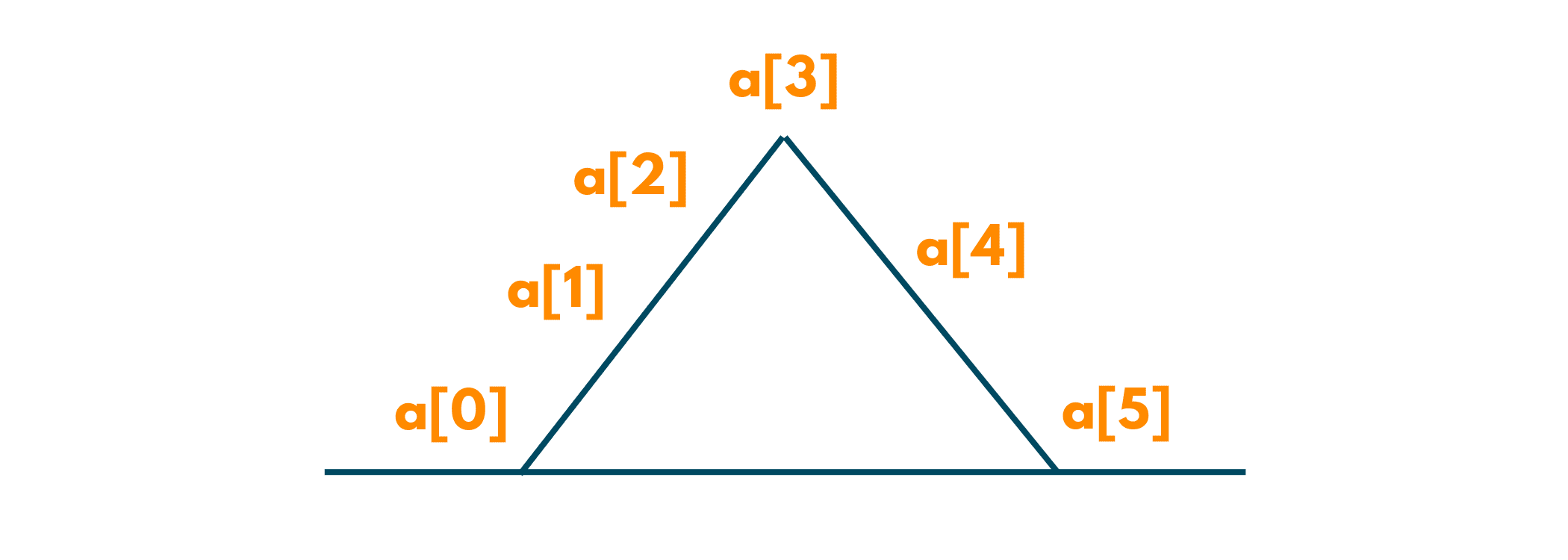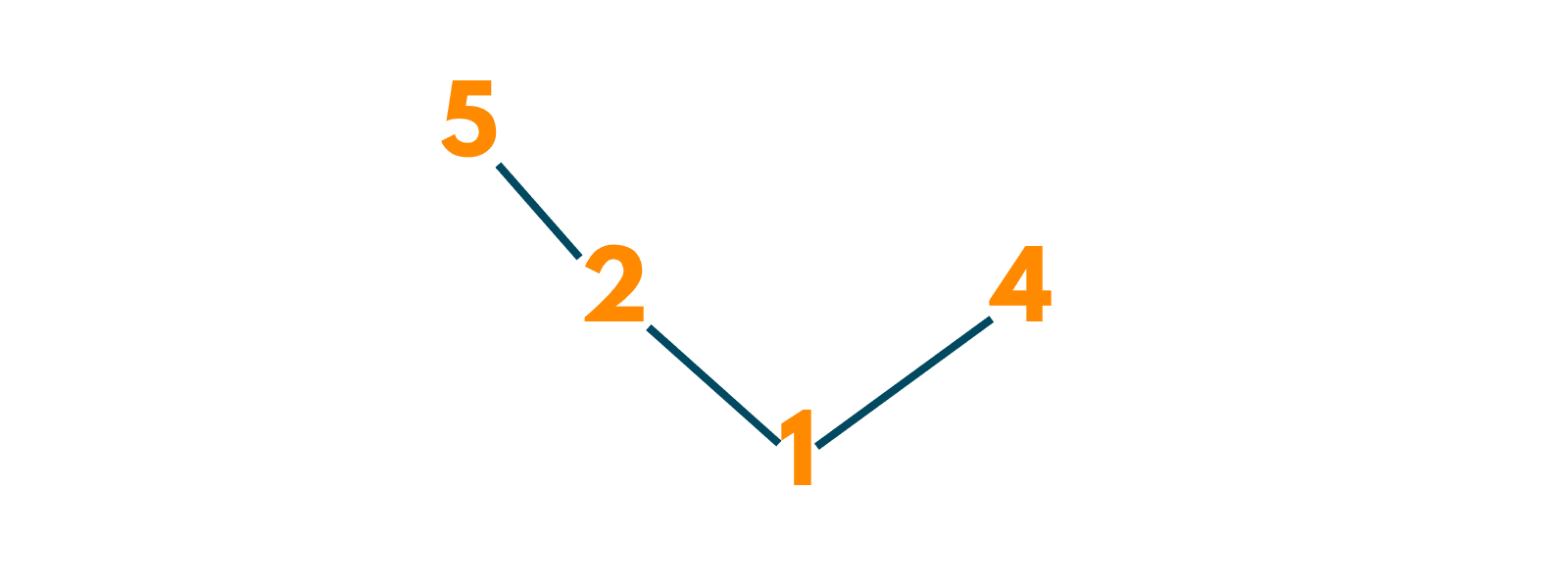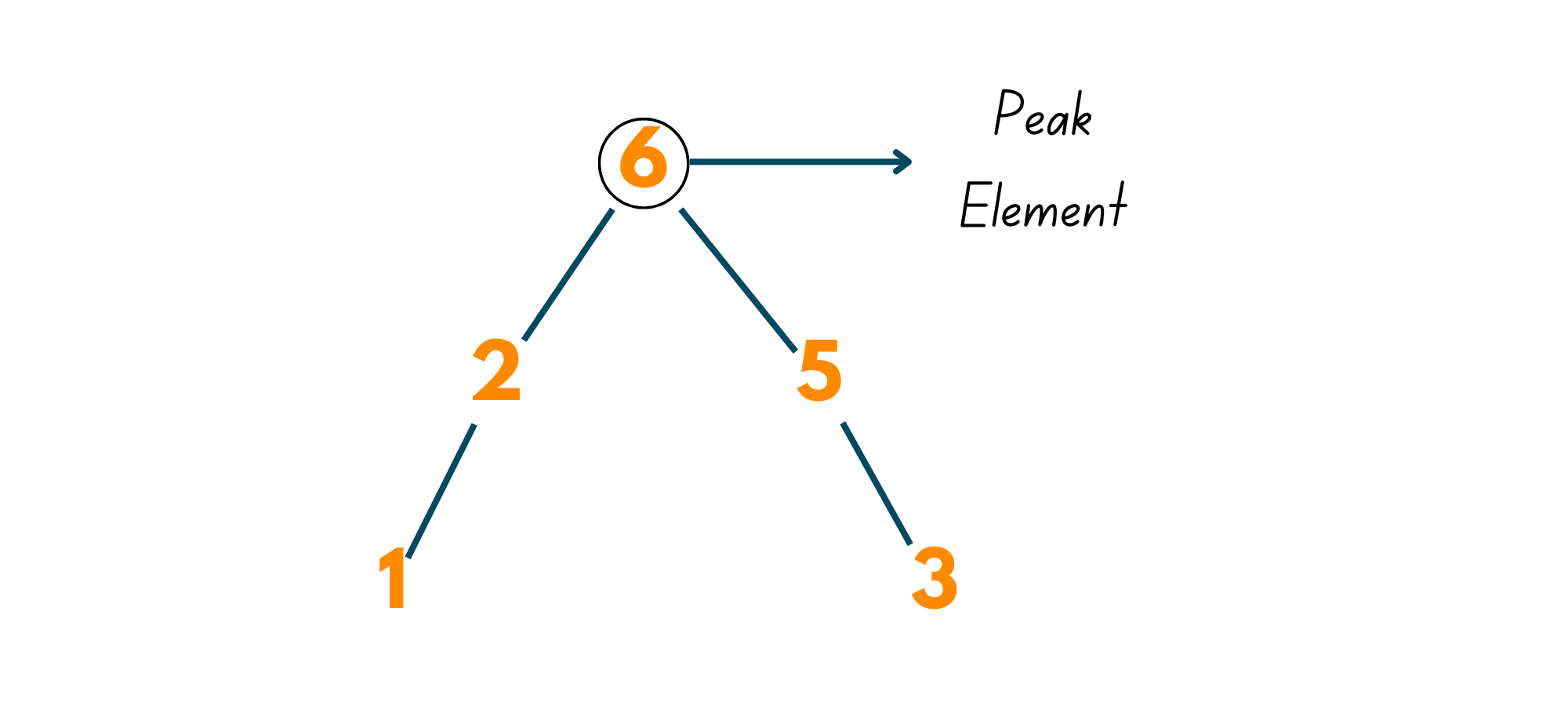# Valid Mountain Array Problem with Solution (C++, Java & Python)

The interviews and hiring challenges have started evolving recently. The companies no longer ask the same old question, but they follow certain trends in their questions by mixing them with real-life objects and puzzles.

So, in today's tech blog we are going to solve a renowned question called Valid Mountain Array. This question has been asked in Google and Amazon and is still a favorite question of hiring recruiters. So, let's go!

## Valid Mountain Array Problem Statement

Here is the problem statement for the Valid Mountain Array: "You are given an array of integers. You need to check whether the given array is a Mountain Array or not."

### What is a Mountain Array?

We will come to the solution eventually, but what if you don't know what a Mountain Array is? Well, then you won't be able to present anything to your interviewer! To save you from such embarrassment, read the whole blog carefully!

A Mountain Array is an array of elements that gradually increase in their first half and later, start decreasing. Think of it as a Mountain! You start climbing its top which is high, you reach the peak and later, descend it in which the height of the mountain keeps on decreasing.

Look at the given image:### Example

Input: [5, 2, 1, 4]

Output: False

Explanation: This is not a Mountain Array. Why? Because here the first element is 5 and after that 2 comes, so the array never actually increased. It keeps on decreasing till its 3rd element and then, again increases. Carefully see the following image:Input: [1, 2, 6, 5, 3]

Output: True

Explanation: This is a valid Mountain Array. Here, firstly the elements are increased from 1 to 2 to 6. Now, 6 is the highest point of this array, from which the array is descending for the rest of its elements. Observe the given illustration:NOTE: The largest element of a Mountain Array is called the Peak Element.

## How to solve Valid Mountain Array?

Now that you have grasped the concept of Mountain Array, let's solve this question using 2 techniques.

### Method 01) Traversing from Left to Right

It is the most basic idea to solve this question. You just start moving from the leftmost integer or we can say, starting off the array. As you traverse, you will keep checking whether the next element is greater than the previous or not.

Now, when you reach a point where you encounter the peak element. You now check whether the next element is less than the previous element or not.

If the above conditions are satisfied, this is a Valid Mountain Array. Else, return False.

### Method 02) Traversing from Opposite Ends

Here, we initialize 2 variables to move from the opposite ends of the array. Now, using a loop we reach the peak element of the array. Similarly, using the loop we reach the peak element from the right side of the array.

Now, in a Mountain Array, you can have only one Peak Element. So, if the peak encountered by the left variable and the right variable is the same, it is a Mountain Array. Otherwise, it is not!

## Code Implementation in C++

Here is the C++ code to solve the Valid Mountain Array problem:

```class Solution {
public:
bool validMountArray(vector<int>& Arr) {
if(Arr.size() < 3)             return false;
int l = 0;
int r = Arr.size() - 1;
while(l + 1 < Arr.size() - 1 && Arr[l] < Arr[l + 1])            l++;
while(r - 1 > 0 && Arr[r] < Arr[r - 1])             r--;
return l == r;
}
};
```

## Code Implementation in Python

Here is the Python code:

```class Solution:
def validMountArray(self, Arr):
if(len(Arr)<3):
return False
i = 1
while(i<len(Arr) and Arr[i]>Arr[i-1]):
i+=1
if(i==1 or i==len(Arr)):
return False
while(i<len(Arr) and Arr[i]<Arr[i-1]):
i+=1
return i==len(Arr)
ob = Solution()
print(ob.validMountArray([0,3,2,1]))
```

## Code Implementation in Java

Here is the Java code:

```class Solution {
public boolean validMountArray(int[] Arr) {
int i = 0;
int j = Arr.length - 1;
int n = Arr.length - 1;
while (i + 1 < n && Arr[i] < Arr[i+1]) {
i++;
}

while (j > 0 && Arr[j] < Arr[j-1]) {
j--;
}

return (i > 0 && i == j && j < n);
}
}
```

Output:

```true
```

## Complexity Analysis

 No. Method Name Time Complexity Space Complexity 1. Traversing from Left to Right O(n) O(1) 2. Traversing from Opposite Ends O(n) O(1)

## Conclusion

This question has come in interviews with tech giants like Google and Amazon. After reading it, we are sure you will be able to solve the Valid Mountain Array problem in the future. So, keep practicing and for more such tech blogs, FavTutor is always there to support you.

### FavTutor - 24x7 Live Coding Help from Expert Tutors!